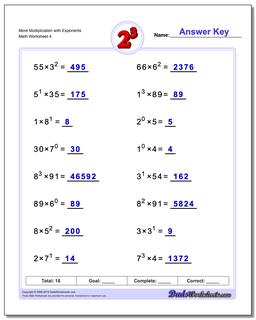# Math Worksheets: Exponents: Exponents: More Multiplication with Exponents (Fourth Worksheet)## More Multiplication with Exponents (Fourth Worksheet)

PropertyValue
DescriptionMore Multiplication with Exponents: Practice exponents worksheets mixing exponents with simple multiplication. Again, these are fantastic exercises for enhancing student comprehension of basic order of operations concepts where exponents are involved. (Fourth Worksheet)
Resource TypeWorksheet